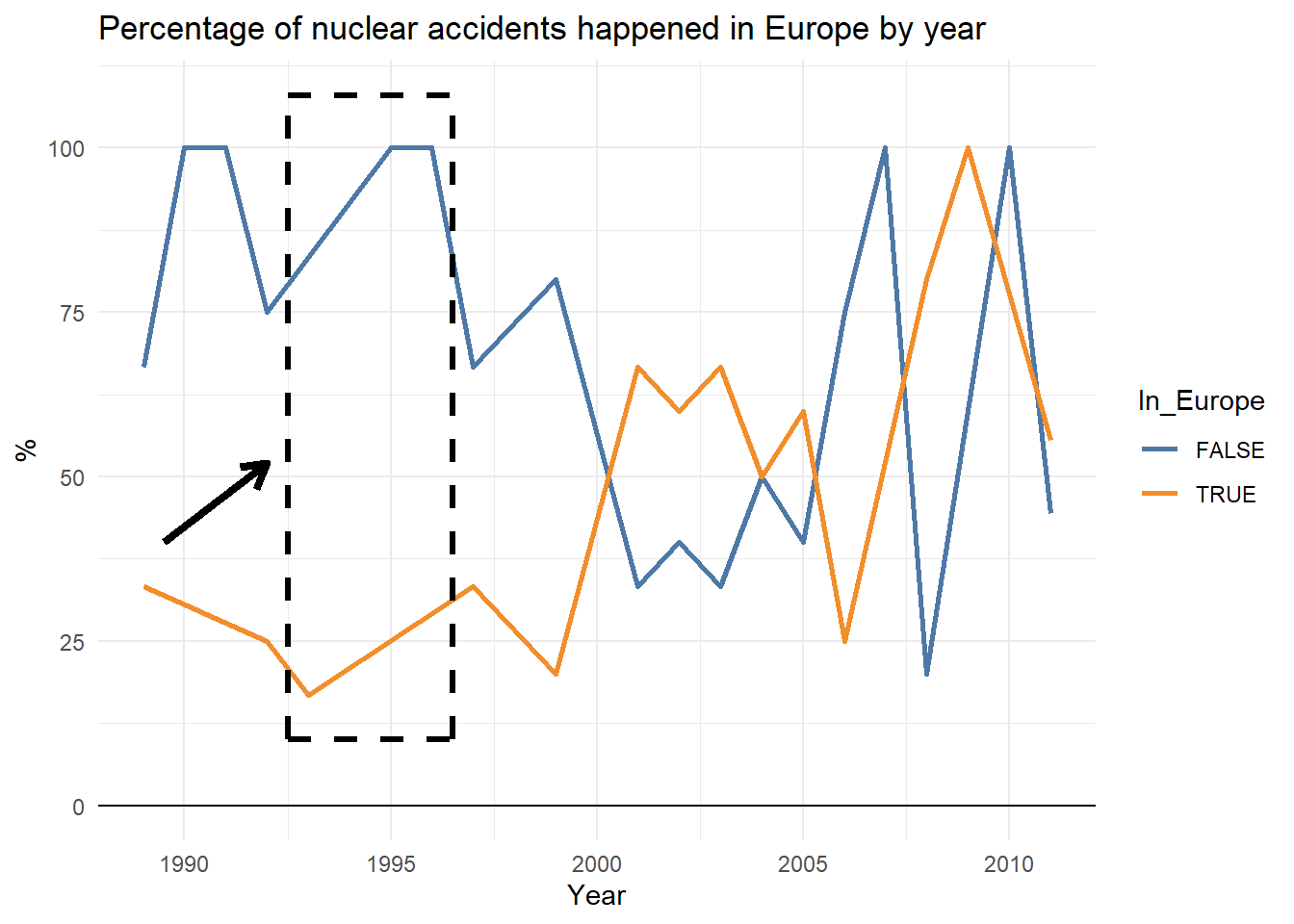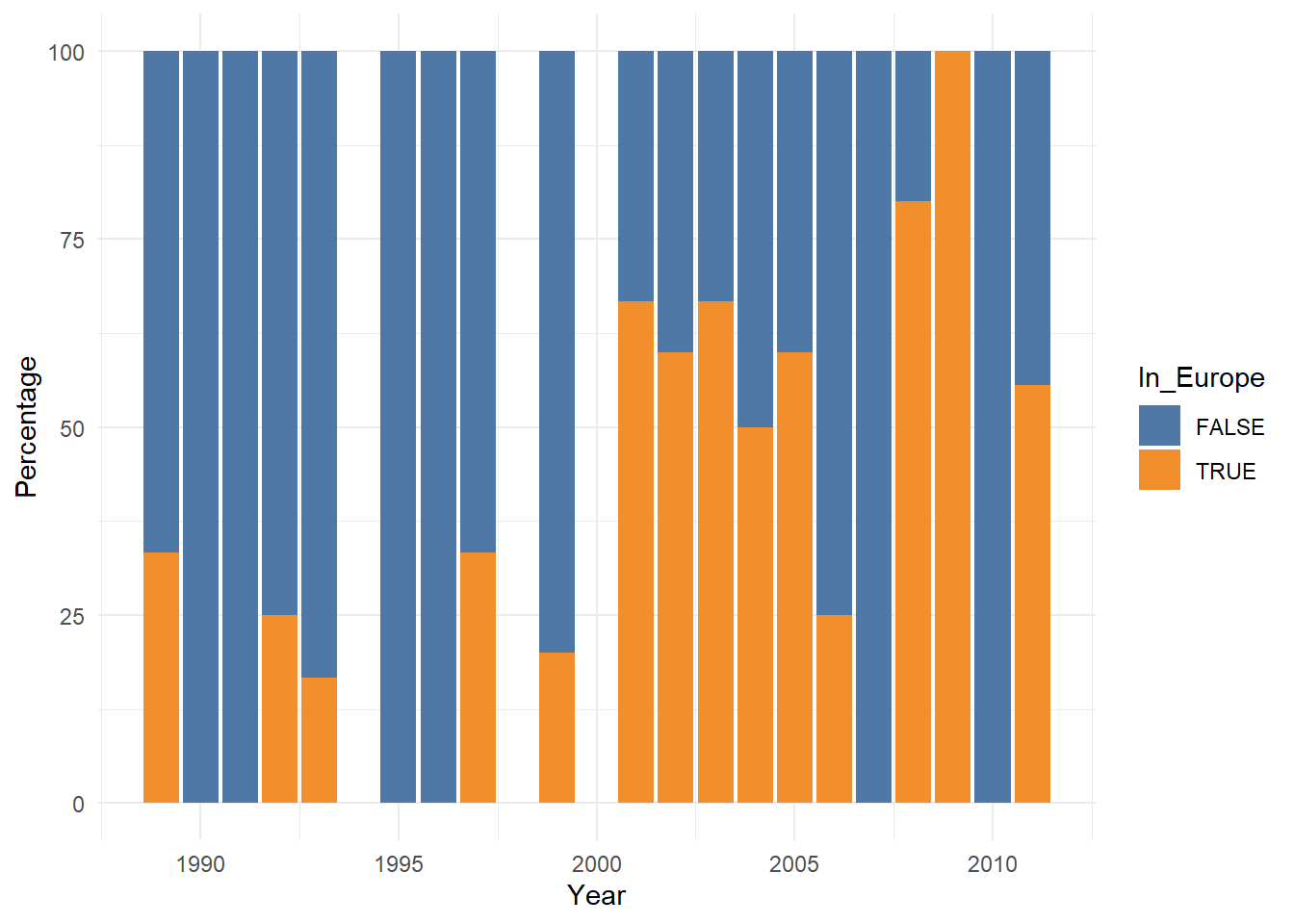Want to share your content on R-bloggers? click here if you have a blog, or here if you don't.

This week I learned about another neat trick with tidyverse functions: the argument `.drop` from the `group_by` function.

To showcase this functionality I made up a simple example with this dataset consisting of nuclear accidents data.

`original_data <- read_csv("https://query.data.world/s/47s7katrhxxd674ulus425k42l5u4s")`

Take a sneak peek of the dataset:

Date Location Cost (millions 2013US\$) INES Smyth Magnitude Region Fatalities
4/26/1986 Kiev, Ukraine 259336 7 8.0 EE 4056
3/11/2011 Fukushima Prefecture, Japan 166089 7 7.5 A 573
12/8/1995 Tsuruga, Japan 15500 NA NA A 0
3/28/1979 Middletown, Pennsylvania, United States 10910 5 7.9 NA 0

Let’s say we are interested in knowing about how does the percentage of accidents happened in Europe vary compared to the rest of the world, and for that reason we simplify the `Region` variable onto the `In_Europe` boolean feature:

```df <- original_data %>%
mutate(Year = Date %>% mdy() %>% year(),
In_Europe = if_else(Region %in% c("EE", "WE"), T, F) %>% as.factor()) %>%
filter(Year %>% between(1989, 2011))```

We then simply compute the percentage of accidents happened every year and plot it

```df_implicitNAs <- df %>%
group_by(Year, In_Europe) %>%
summarize(N = n()) %>%
mutate(Percentage = round(N / sum(N) * 100, 1)) ```

If that chunk puzzles you, I explain what is going on under the hood in this post.

In the plot below I highlighted the strange result I found: being that there are only two possibilities (the accident happened in Europe or not) the sum of the ratios should add up to 100, right?Not a beautiful plot but aesthetic considerations apart, what is going on here? Did we fail with the maths? Not exactly. This result is due to a tricky behaviour of the `summarise` function related with the missing values.

If we take a look to the first rows, we can see how in 1990 and 1991 there weren’t any nuclear accident in Europe, but that information is implicit instead of explicit.

Year In_Europe N Percentage
1989 FALSE 4 66.7
1989 TRUE 2 33.3
1990 FALSE 1 100.0
1991 FALSE 3 100.0
1992 FALSE 3 75.0
1992 TRUE 1 25.0

When plotting with the line graph, ggplot is connecting the data points between 1989 and 1992 and therefore displaying misleading information. Obviously this is not ggplot’s fault, it’s simply how it works.

We could easily solve this problem chossing an more suitable graph as we will see below, but this example is still useful to learn about the `.drop` argument: The default behaviour of the `group_by` function is to drop zero-length groups, and therefore it’s making implicit that piece of information. We can override the default behaviour simply adding `.drop = FALSE` to the call:

```df_explicitNAs <- df %>%
group_by(Year, In_Europe, .drop = FALSE) %>%
summarize(N = n()) %>%
mutate(Percentage = round(N / sum(N) * 100, 1))```

Now we can see the years when there were no accidents in Europe:

Year In_Europe N Percentage
1989 FALSE 4 66.7
1989 TRUE 2 33.3
1990 FALSE 1 100.0
1990 TRUE 0 0.0
1991 FALSE 3 100.0
1991 TRUE 0 0.0

And now the graph doesn’t deceive us anymore:Let’s face, however, that we could have tackled this problem choosing a more suitable geom. Whenever you need to display any normalized variable, using `geom_col()` is usually a better approach: